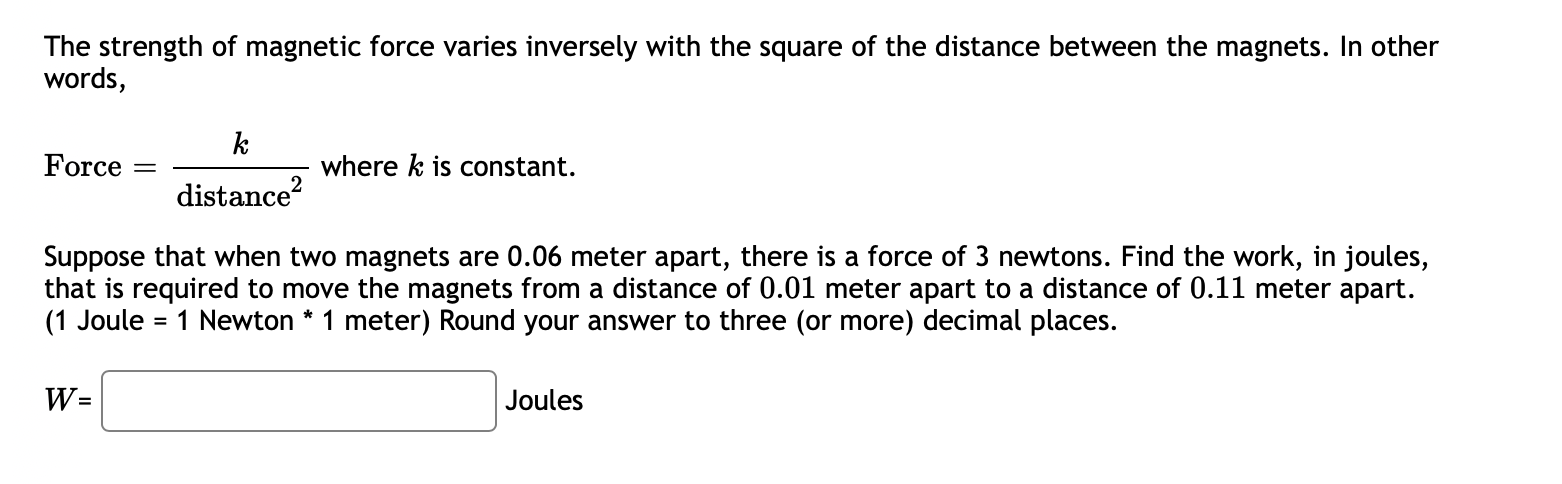# Question The strength of magnetic force varies inversely with the square of the distance between the magnets. In other words, Force = k distance where k is constant. Suppose that when two magnets are 0.06 meter apart, there is a force of 3 newtons. Find the work, in joules, that is required to move the magnets from a distance of 0.01 meter apart to a distance of 0.11 meter apart. (1 Joule = 1 Newton * 1 meter) Round your answer to three (or more) decimal places. W= Joules